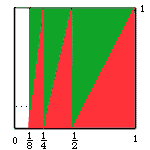Date: Fri, 28 Nov 1997 07:24:00 -0600 (CST)

Name: Rosa

Level: Secondary

Question:
There is a figure, it has unlimited perimeter but has limited area , what is the figure and how to draw it ?

Thank you very much!

Hi Rosa

 Take the unit square, mark the base at 1/2, 1/4, 1/8, 1/16 ... and construct triangles as in the diagram. The region shaded red has the same area as the region shaded green and together they cover the square. Hence the area of the red region is 1/2. The perimeter of the red region is the sum of the perimeters of the red triangles and each triangle has one side of length 1. Thus the perimeter of the red region is larger than 1+1+1+1+... which is unbounded.Cheers
Harley

Go to Math Central